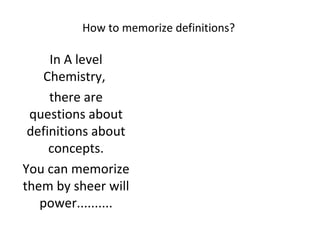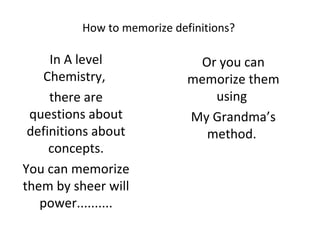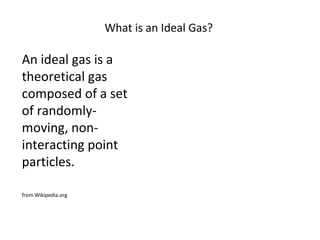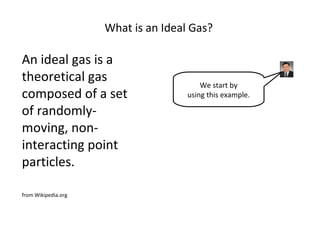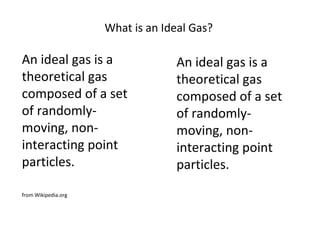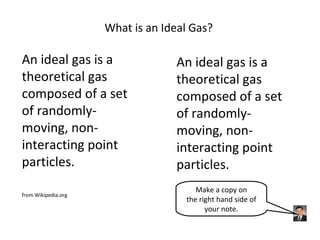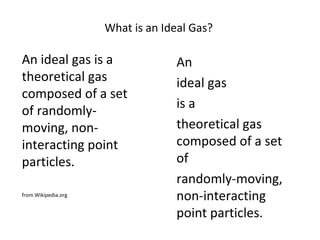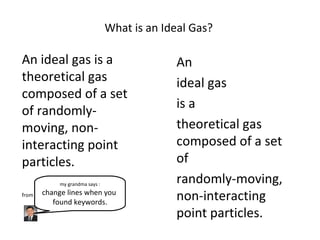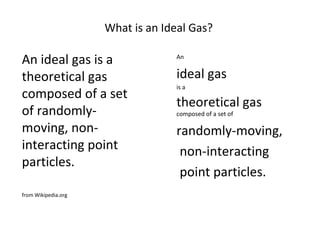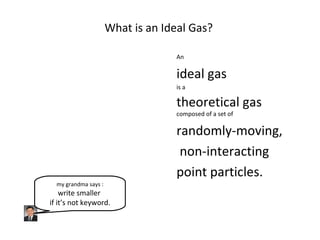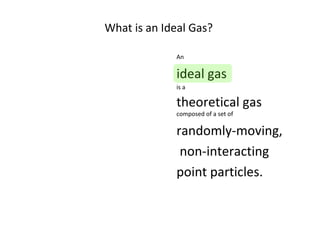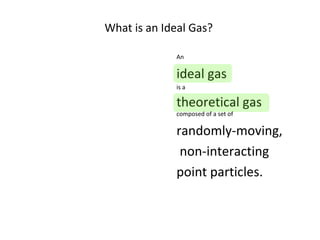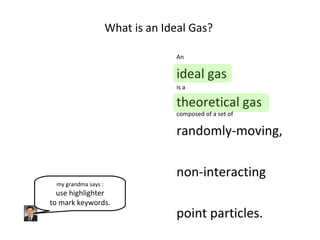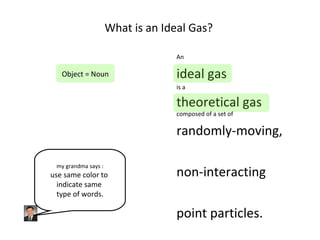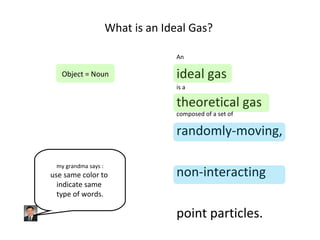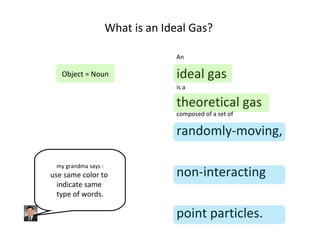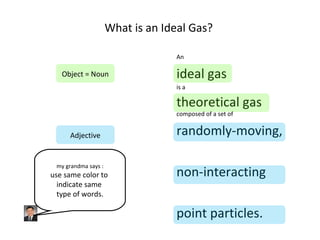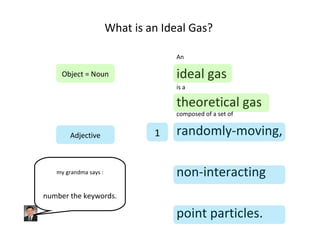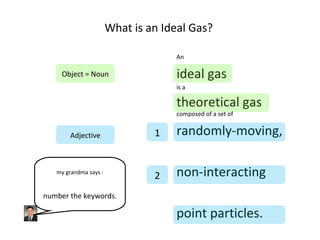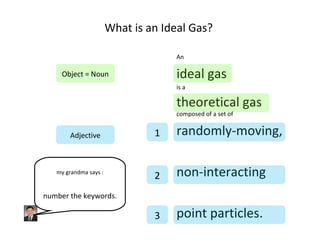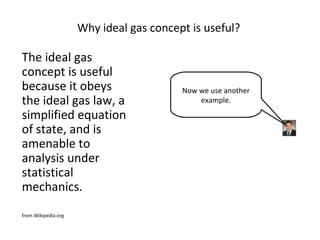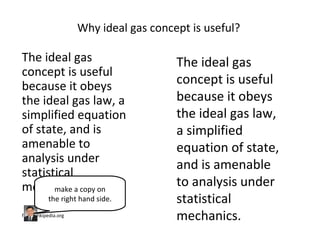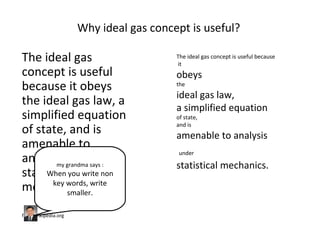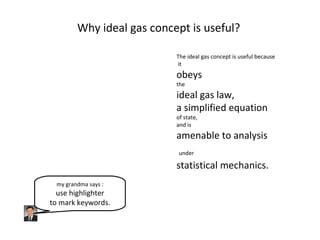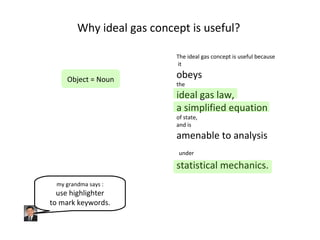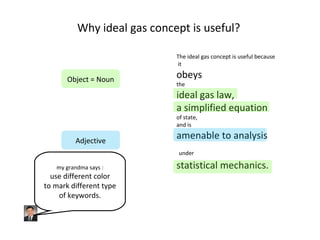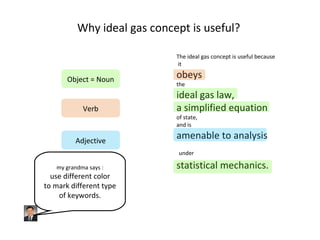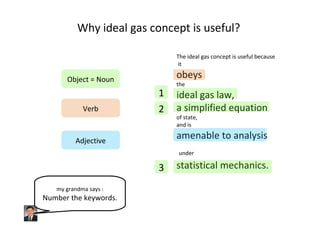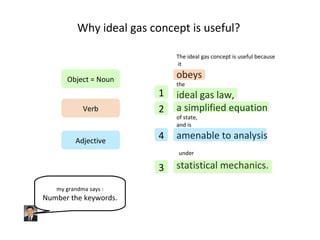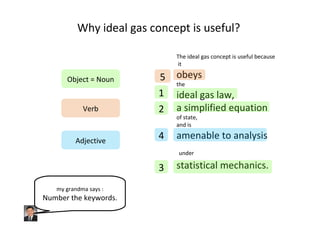1 of 32

### Definitions ideal gas v004

1. How to memorize definitions? In A level Chemistry, there are questions about definitions about concepts. You can memorize them by sheer will power..........
2. How to memorize definitions? In A level Chemistry, there are questions about definitions about concepts. You can memorize them by sheer will power.......... Or you can memorize them using My Grandma’s method.
3. What is an Ideal Gas? An ideal gas is a theoretical gas composed of a set of randomly-moving, non-interacting point particles. from Wikipedia.org
4. What is an Ideal Gas? An ideal gas is a theoretical gas composed of a set of randomly-moving, non-interacting point particles. from Wikipedia.org We start by using this example.
5. What is an Ideal Gas? An ideal gas is a theoretical gas composed of a set of randomly-moving, non-interacting point particles. from Wikipedia.org An ideal gas is a theoretical gas composed of a set of randomly-moving, non-interacting point particles.
6. What is an Ideal Gas? An ideal gas is a theoretical gas composed of a set of randomly-moving, non-interacting point particles. from Wikipedia.org An ideal gas is a theoretical gas composed of a set of randomly-moving, non-interacting point particles. Make a copy on the right hand side of your note.
7. What is an Ideal Gas? An ideal gas is a theoretical gas composed of a set of randomly-moving, non-interacting point particles. from Wikipedia.org An ideal gas is a theoretical gas composed of a set of randomly-moving, non-interacting point particles.
8. What is an Ideal Gas? An ideal gas is a theoretical gas composed of a set of randomly-moving, non-interacting point particles. from Wikipedia.org An ideal gas is a theoretical gas composed of a set of randomly-moving, non-interacting point particles. my grandma says : change lines when you found keywords.
9. What is an Ideal Gas? An ideal gas is a theoretical gas composed of a set of randomly-moving, non-interacting point particles. from Wikipedia.org An ideal gas is a theoretical gas composed of a set of randomly-moving, non-interacting point particles.
10. What is an Ideal Gas? An ideal gas is a theoretical gas composed of a set of randomly-moving, non-interacting point particles. my grandma says : write smaller if it’s not keyword.
11. What is an Ideal Gas? An ideal gas is a theoretical gas composed of a set of randomly-moving, non-interacting point particles.
12. What is an Ideal Gas? An ideal gas is a theoretical gas composed of a set of randomly-moving, non-interacting point particles.
13. What is an Ideal Gas? An ideal gas is a theoretical gas composed of a set of randomly-moving, non-interacting point particles. my grandma says : use highlighter to mark keywords.
14. What is an Ideal Gas? An ideal gas is a theoretical gas composed of a set of randomly-moving, non-interacting point particles. Object = Noun my grandma says : use same color to indicate same type of words.
15. What is an Ideal Gas? An ideal gas is a theoretical gas composed of a set of randomly-moving, non-interacting point particles. Object = Noun my grandma says : use same color to indicate same type of words.
16. What is an Ideal Gas? An ideal gas is a theoretical gas composed of a set of randomly-moving, non-interacting point particles. Object = Noun my grandma says : use same color to indicate same type of words.
17. What is an Ideal Gas? An ideal gas is a theoretical gas composed of a set of randomly-moving, non-interacting point particles. Object = Noun my grandma says : use same color to indicate same type of words.
18. What is an Ideal Gas? An ideal gas is a theoretical gas composed of a set of randomly-moving, non-interacting point particles. Object = Noun Adjective my grandma says : use same color to indicate same type of words.
19. What is an Ideal Gas? An ideal gas is a theoretical gas composed of a set of randomly-moving, non-interacting point particles. Object = Noun Adjective 1 my grandma says : number the keywords.
20. What is an Ideal Gas? An ideal gas is a theoretical gas composed of a set of randomly-moving, non-interacting point particles. Object = Noun Adjective 1 2 my grandma says : number the keywords.
21. What is an Ideal Gas? An ideal gas is a theoretical gas composed of a set of randomly-moving, non-interacting point particles. Object = Noun Adjective 1 2 3 my grandma says : number the keywords.
22. Why ideal gas concept is useful? The ideal gas concept is useful because it obeys the ideal gas law, a simplified equation of state, and is amenable to analysis under statistical mechanics. from Wikipedia.org Now we use another example.
23. Why ideal gas concept is useful? The ideal gas concept is useful because it obeys the ideal gas law, a simplified equation of state, and is amenable to analysis under statistical mechanics. from Wikipedia.org The ideal gas concept is useful because it obeys the ideal gas law, a simplified equation of state, and is amenable to analysis under statistical mechanics. make a copy on the right hand side.
24. Why ideal gas concept is useful? The ideal gas concept is useful because it obeys the ideal gas law, a simplified equation of state, and is amenable to analysis under statistical mechanics. from Wikipedia.org The ideal gas concept is useful because it obeys the ideal gas law, a simplified equation of state, and is amenable to analysis under statistical mechanics. my grandma says : When you write non key words, write smaller.
25. Why ideal gas concept is useful? The ideal gas concept is useful because it obeys the ideal gas law, a simplified equation of state, and is amenable to analysis under statistical mechanics. my grandma says : use highlighter to mark keywords.
26. Why ideal gas concept is useful? The ideal gas concept is useful because it obeys the ideal gas law, a simplified equation of state, and is amenable to analysis under statistical mechanics. Object = Noun my grandma says : use highlighter to mark keywords.
27. Why ideal gas concept is useful? The ideal gas concept is useful because it obeys the ideal gas law, a simplified equation of state, and is amenable to analysis under statistical mechanics. Object = Noun Adjective my grandma says : use different color to mark different type of keywords.
28. Why ideal gas concept is useful? The ideal gas concept is useful because it obeys the ideal gas law, a simplified equation of state, and is amenable to analysis under statistical mechanics. Object = Noun Adjective Verb my grandma says : use different color to mark different type of keywords.
29. Why ideal gas concept is useful? The ideal gas concept is useful because it obeys the ideal gas law, a simplified equation of state, and is amenable to analysis under statistical mechanics. Object = Noun Adjective Verb 1 2 3 my grandma says : Number the keywords.
30. Why ideal gas concept is useful? The ideal gas concept is useful because it obeys the ideal gas law, a simplified equation of state, and is amenable to analysis under statistical mechanics. Object = Noun Adjective Verb 4 1 2 3 my grandma says : Number the keywords.
31. Why ideal gas concept is useful? The ideal gas concept is useful because it obeys the ideal gas law, a simplified equation of state, and is amenable to analysis under statistical mechanics. Object = Noun Adjective Verb 4 1 5 2 3 my grandma says : Number the keywords.
32. Why ideal gas concept is useful? The ideal gas concept is useful because it obeys the ideal gas law, a simplified equation of state, and is amenable to analysis under statistical mechanics. Object = Noun Adjective Verb 4 1 5 2 3 my grandma says : Number the keywords.